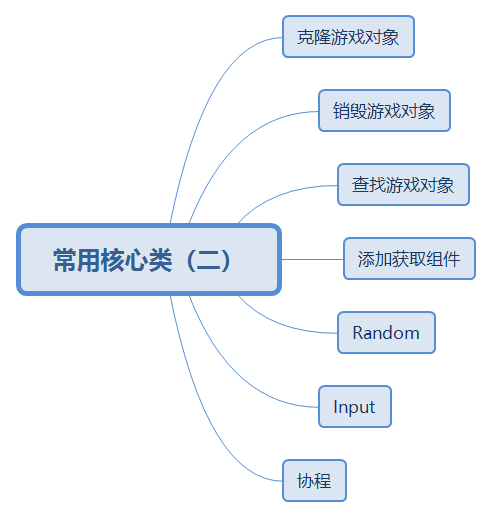WHCSRL 技术网

# 常用的核心类（二）//在预设体的位置克隆游戏对象

GameObject go1=Instantiate(cube);

//在固定位置克隆游戏对象

//Quaternion.identity 游戏对象不旋转：Quaternion(0,0,0,0)

GameObject go2 =Instantiate(cube,new Vector3(0,0,5),Quaternion.identity);

Destroy(go1);    //直接销毁对象go1

Destroy(go2,3);   //停3秒后销毁对象go2

GameObject  cube1=GameObject.Find("Player");

GameObject  cube2=GameObject.FindWithTag("Player");

//1、把Move这个脚本加到cube这个游戏对象上

//2、给游戏物体添加刚体

//3、给游戏物体添加球体碰撞器

//1、获取脚本组件Move

Move m=cube.GetComponent<Move>();

//2、获取刚体组件

Rigidbody r=cube.GetComponent<Rigidbody>();

float a=Random.value;         //返回0.0（包括）到1.0（包括）之间的数。
int b=Random.Range(0,100) ;         //包括最小但不包括最大

float c=Random.Range(0.0f,5.5f); //包括最大和最小

1. 鼠标事件

GetMouseButton(0):按下鼠标左键不动，程序会一直运行，松开左键程序停止运行。

GetMouseButton(2):按下鼠标中键不动，程序会一直运行，松开中键程序停止运行。

GetMouseButton(1):按下鼠标右键不动，程序会一直运行，松开右键程序停止运行。

GetMouseButtonDown(0):按下鼠标左键时，程序运行一次

GetMouseButtonDown(1):按下鼠标右键时，程序运行一次

GetMouseButtonUp(2):按下鼠标中键时，程序不运行，松开中键时，程序运行一次。

if(Input.GetMouseButton(0)){

执行语句;

}

1. 键盘事件

GetKey     当通过名称指定的按键被用户按住时返回true

GetKeyDown   当用户按下指定名称的按键时的那一帧返回true。

GetKeyUp    在用户释放给定名字的按键的那一帧返回true。

GetAxis（"Horizontal"）和GetAxis（"Vertical"）    水平轴和垂直轴

if(Input.GetKey("Down")){

执行语句;

}

float speed = 3f;

void Update () {

//float h = Time.deltaTime * speed;

/*if (Input.GetKey(KeyCode.A))

{

transform.Translate(new Vector3(-h, 0, 0));

}

if (Input.GetKey(KeyCode.D))

{

transform.Translate(new Vector3(h, 0, 0));

}

if (Input.GetKey(KeyCode.W))

{

transform.Translate(new Vector3(0, 0, h));

}

if (Input.GetKey(KeyCode.S))

{

transform.Translate(new Vector3(0, 0, -h));

}*/

//Horizontal

//Vertical

float h= Input.GetAxis("Horizontal")*Time.deltaTime*speed;

float v = Input.GetAxis("Vertical") * Time.deltaTime * speed;

//transform.Translate(h, 0, v);

transform.Translate(new Vector3(h, 0, v));

}

1. 自定义按钮

GetButton   根据按钮名称返回true当对应的虚拟按钮被按住时。

GetButtonDown  在给定名称的虚拟按钮被按下的那一帧返回true。

GetButtonUp    在用户释放指定名称的虚拟按钮时返回true。

if (Input.GetButtonDown("Fire1"))

{

GameObject go = Instantiate(cube);

Rigidbody r = go.GetComponent<Rigidbody>();//从预设体身上获取刚体组件

}

Yield语句是一种特殊类型的Return（返回）语句，它可以确保函数在下一次被执行时，不是从头开始，而是从Yield语句处开始。

public GameObject cube;

void Start () {

StartCoroutine("NewCube");

}

IEnumerator NewCube()

{

while (true)

{

Instantiate(cube);

yield return new WaitForSeconds(3f);

}

}